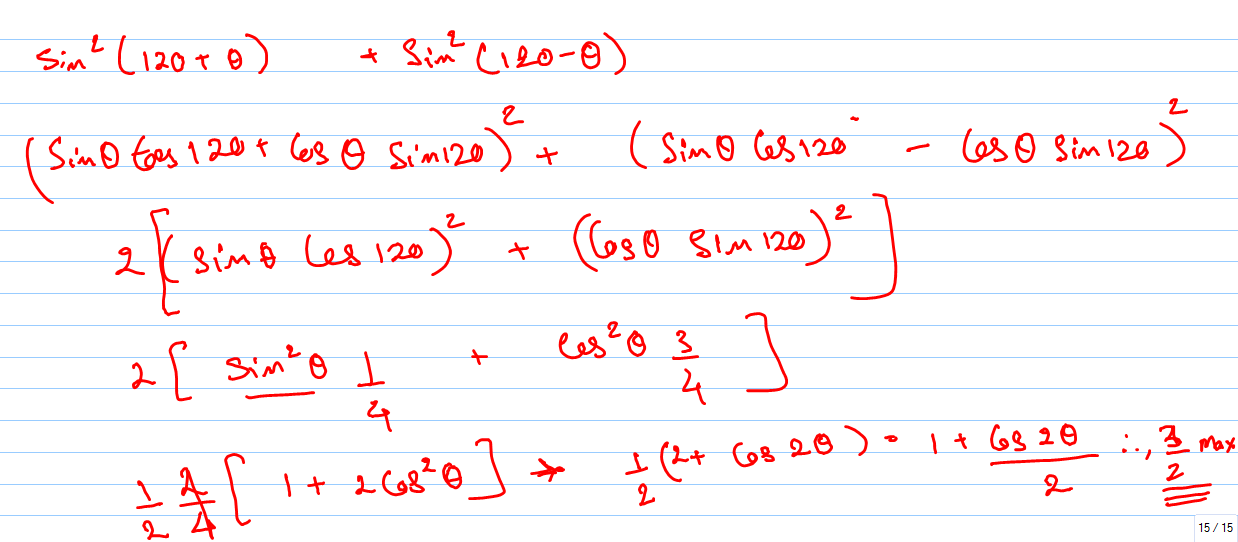Click to Chat

1800-1023-196

+91-120-4616500

CART 0

• 0

MY CART (5)

Use Coupon: CART20 and get 20% off on all online Study Material

ITEM
DETAILS
MRP
DISCOUNT
FINAL PRICE
Total Price: Rs.

There are no items in this cart.
Continue Shopping
`        MAXIMUM value of sin^2(120+theta)+sun^2(120-theta) is `
one year agoSaurabh Koranglekar
3161 Points
``````
one year ago
```							sin(t)^2 = (1/2) * (1 - cos(2t)) sin(120 + a)^2 + sin(120 - a)^2 => (1/2) * (1 - cos(2 * (120 + a))) + (1/2) * (1 - cos(2 * (120 - a))) => (1/2) * (1 - cos(240 + 2a) + 1 - cos(240 - 2a)) => (1/2) * (2 - (cos(240 + 2a) + cos(240 - 2a))) => 1 - (1/2) * (cos(240)cos(2a) - sin(240)sin(2a) + cos(240)cos(2a) + sin(240)sin(2a)) => 1 - (1/2) * (2 * cos(240) * cos(2a)) => 1 - cos(240) * cos(2a) cos(t) = 1 when t = 360 * k cos(t) = -1 when t = 180 + 360 * k 1 - cos(240) * (-1) => 1 + cos(240) => 1 - 1/2 => 1/2 1 - cos(240) * 1 => 1 - (-1/2) => 3/2 3/2 is the maximum
```
one year ago
Think You Can Provide A Better Answer ?

## Other Related Questions on Trigonometry

View all Questions »### Course Features

• 731 Video Lectures
• Revision Notes
• Previous Year Papers
• Mind Map
• Study Planner
• NCERT Solutions
• Discussion Forum
• Test paper with Video Solution### Course Features

• 31 Video Lectures
• Revision Notes
• Test paper with Video Solution
• Mind Map
• Study Planner
• NCERT Solutions
• Discussion Forum
• Previous Year Exam Questions